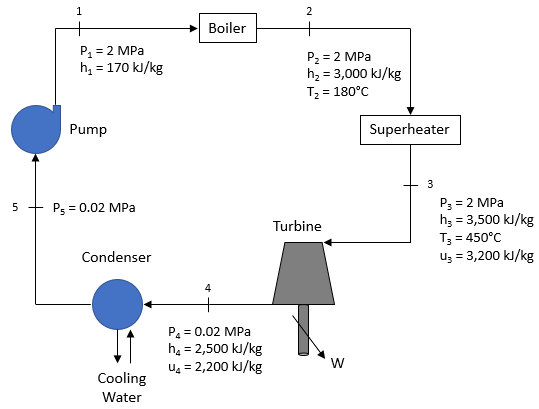## Rankine Cycle

The Rankine cycle below is used to power a plant complex with water as the working fluid. If the pump’s isentropic efficiency is 75% and the fluid’s mass flow rate is 25 kg/s, how much power (kW) is required to drive the pump? Assume steady state and steady flow conditions. Also, disregard pressure losses and kinetic/potential energy effects. Note the density of water is 1,000 kg/m^3.Hint
The isentropic efficiency for a pump is
$$\eta_{pump}=\frac{w_s}{w_{actual}}$$$where $$w_s$$ is the isentropic pump work per unit mass and $$w_{actual}$$ is the actual pump work per unit mass. Hint 2 The isentropic pump work for a constant density fluid is $$w_{s}=v(\Delta P)$$$
where $$v$$ is the specific volume of the working fluid and $$\Delta P$$ is the change in pressure.
The isentropic pump work for a constant density fluid is
$$w_{s}=v(P_1-P_5)$$$where $$v$$ is the specific volume of the working fluid and $$\Delta P$$ is the change in pressure. Since $$v=\rho^{-1}$$ where $$\rho$$ is the density: $$w_{s}=\frac{1(m^3)}{1,000kg}(2,000kPa-20kPa)=1.98\:kJ/kg$$$
The isentropic efficiency for a pump is
$$\eta_{pump}=\frac{w_s}{w_{actual}}$$$where $$w_s$$ is the isentropic pump work per unit mass and $$w_{actual}$$ is the actual pump work per unit mass. $$w_{actual}=\frac{w_s}{\eta_{pump}}=\frac{1.98kJ/kg}{0.75}=2.64\:kJ/kg$$$
Thus, to get the actual power required by the pump:
$$\dot{W}_{actual}=w_{actual}\times\dot{m}=2.64\frac{kJ}{kg}\times 25\frac{kg}{s}=66\:kW$$\$
66 kW